# 质子交换膜电解池两相传热传质瞬态响应二维数值模拟Two-Dimensional Numerical Simulation of Transient Response of Two-Phase Heat and Mass Transfer in a Proton Exchange Membrane Electrolyzer Cell

DOI: 10.12677/SE.2018.81002, PDF, HTML, XML, 下载: 902  浏览: 3,061  国家自然科学基金支持

Abstract: A two-dimensional, two-phase flow, transient, and non-isothermal model is developed to study the transient characteristics in a proton exchange membrane electrolyzer cell. The transient response of liquid water, gas species, temperature, and current density was investigated. Simulation results indicate that the transient response mechanisms of liquid water saturation, temperature, current density, and gas mass fraction are different. The response time of liquid water from transient to steady state is longer than that of temperature, current density, and gas mass fraction. The gradient of liquid water saturation in porous medium is larger than that in the flow channel. The gradient of liquid water saturation near the inlet section of gas flow channel is less than that near the outlet section of gas flow channel. In the electrolyzer cell mode, the cell temperature is decreased and the lowest temperature always appears in the anode catalyst layer. The temperature difference of the entire cell is less than 3 K.

1. 引言

PEM电解池的反应物和生成物均对环境无污染，同时还具有启动速度快、可再生、电流密度高等优点，使其具有广泛的应用前景   。此外，电解池与燃料电池组合而成的一体式再生燃料电池因其具有很高的比能量和比功率，在民用、航空航天及偏远地区的储能系统等领域内得到了较为广泛的应用    。

2. 模型描述

2.1. 模型的计算区域及假设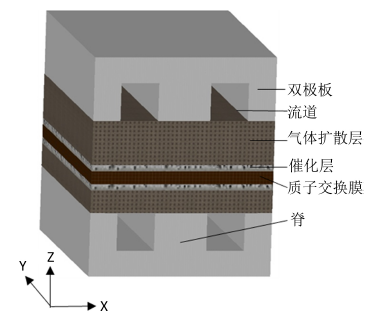Figure 1. Schematic diagram of a PEM electrolyzer cell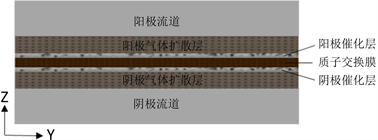Figure 2. Computational domain of 2D model

2.2. 控制方程

2.2.1. 电荷守恒

$\nabla \cdot {i}_{\text{s}}=\nabla \cdot \left(-{\sigma }_{\text{s}}^{\text{eff}}\nabla {\varphi }_{\text{s}}\right)={S}_{{\varphi }_{\text{s}}}$ (1)

$\nabla \cdot {i}_{\text{m}}=\nabla \cdot \left(-{\sigma }_{\text{m}}^{\text{eff}}\nabla {\varphi }_{\text{m}}\right)={\text{S}}_{{\varphi }_{\text{m}}}$ (2)

${i}_{\text{c}}={\alpha }_{\text{v}}{i}_{\text{c,ref}}{\left(\frac{{C}_{{\text{H}}_{\text{2}}}}{{C}_{{\text{H}}_{\text{2,ref}}}}\right)}^{\frac{1}{2}}\left(\mathrm{exp}\left(\frac{{\alpha }_{\text{a}}F{\eta }_{\text{a}}}{RT}\right)-\mathrm{exp}\left(\frac{-{\alpha }_{\text{c}}F{\eta }_{\text{c}}}{RT}\right)\right)$ (3)

${i}_{\text{a}}={\alpha }_{\text{v}}{i}_{\text{a,ref}}\left(\frac{{C}_{{\text{O}}_{\text{2}}}}{{C}_{{\text{O}}_{\text{2,ref}}}}\right)\left(\mathrm{exp}\left(\frac{{\alpha }_{\text{a}}F{\eta }_{\text{a}}}{RT}\right)-\mathrm{exp}\left(\frac{-{\alpha }_{\text{c}}F{\eta }_{\text{c}}}{RT}\right)\right)$ (4)

${\eta }_{\text{c}}={\varphi }_{\text{s}}-{\varphi }_{\text{m}}$ (5)

${\eta }_{\text{a}}={\varphi }_{\text{s}}-{\varphi }_{\text{m}}-{E}_{\text{eq}}$ (6)

${E}_{\text{eq}}=1.229-9×{10}^{-4}\left(T-298.15\right)$ (7)

2.2.2. 质量和动量的守恒

$\frac{\partial \left({\epsilon }_{\text{g}}^{\text{eff}}{\rho }_{\text{g}}\right)}{\partial t}+\nabla \cdot \left({\rho }_{\text{g}}{u}_{\text{g}}\right)={\text{S}}_{\text{m}}$ (8)

$\frac{{\rho }_{\text{g}}}{{\epsilon }_{\text{g}}^{\text{eff}}}\left(\frac{\partial {u}_{\text{g}}}{\partial t}+\left({u}_{\text{g}}\cdot \nabla \right)\frac{{u}_{\text{g}}}{{\epsilon }_{\text{g}}^{\text{eff}}}\right)=\nabla \cdot \left[-pl+\frac{\mu }{{\epsilon }_{\text{g}}^{\text{eff}}}\left(\nabla {u}_{g}+{\left(\nabla {u}_{g}\right)}^{\text{T}}\right)-\frac{2\mu }{3{\epsilon }_{\text{g}}^{\text{eff}}}\left(\nabla \cdot {u}_{\text{g}}\right)l\right]-\left(\frac{\mu }{k}+\frac{{\text{S}}_{\text{m}}}{{\epsilon }_{\text{g}}^{\text{eff}}{}^{{}^{2}}}\right){u}_{\text{g}}+F$ (9)

${\epsilon }_{\text{g}}^{\text{eff}}={\epsilon }_{0}\left(1-s\right)$ (10)

2.2.3. 组分守恒

$\begin{array}{l}{\rho }_{\text{g}}\frac{\partial {\omega }_{i}}{\partial t}-\nabla \cdot \left({\rho }_{g}{\omega }_{i}\sum {D}_{ik}^{\text{eff}}\left(\nabla {x}_{k}+\frac{1}{p}\left[\left({x}_{k}-{\omega }_{k}\right)\nabla p\right]\right)+{D}_{i}^{\text{T}}\frac{\nabla T}{T}\right)\\ +\text{\hspace{0.17em}}{\rho }_{\text{g}}\left({u}_{\text{g}}\cdot \nabla \right){\omega }_{i}={S}_{i}\end{array}$ (11)

${x}_{k}=\frac{{\omega }_{k}}{{M}_{k}}{M}_{n},\text{\hspace{0.17em}}\text{\hspace{0.17em}}{M}_{n}={\left(\underset{i}{\sum }\frac{{\omega }_{i}}{{M}_{i}}\right)}^{-1}$ (12)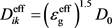(13)

2.2.4. 液态水传输的守恒方程

$\frac{\partial \left(\epsilon {\rho }_{l}\right)}{\partial t}+\nabla \cdot \left({\rho }_{l}{u}_{l}\right)={S}_{l}$ (14)

${p}_{\text{c}}={p}_{\text{l}}-{p}_{\text{g}}=f\left(s\right)$ (15)

${u}_{\text{g}}=-\frac{{K}_{\text{g}}}{{\mu }_{\text{g}}}\nabla {p}_{\text{g}}$ (16)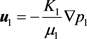(17)

${p}_{\text{c}}=\sigma \mathrm{cos}\theta {\left(\frac{\epsilon }{{K}_{0}}\right)}^{\frac{1}{2}}J\left(s\right)$ (18)

$J\left(s\right)=\left\{\begin{array}{ll}1.417\left(1-s\right)-2.12{\left(1-s\right)}^{2}+1.263{\left(1-s\right)}^{3},\hfill & 0<\theta <{90}^{\circ }\hfill \\ 1.417s-2.12{s}^{2}+1.263{s}^{3},\hfill & {90}^{\circ }<\theta <{180}^{\circ }\hfill \end{array}$ (19)

${K}_{\text{g}}={K}_{0}{\left(1-s\right)}^{3},\text{\hspace{0.17em}}\text{\hspace{0.17em}}{K}_{\text{l}}={K}_{0}{s}^{3}$ (20)

$\frac{\partial \left(\epsilon {\rho }_{\text{l}}s\right)}{\partial t}+\nabla \cdot \left(-\frac{{\rho }_{\text{l}}{K}_{\text{l}}}{{\mu }_{\text{l}}}\frac{\text{d}{p}_{\text{c}}}{\text{d}s}\right)\nabla s+\nabla \cdot \frac{{\rho }_{\text{l}}{\mu }_{\text{g}}{K}_{\text{l}}}{{\mu }_{\text{l}}{K}_{\text{g}}}{u}_{g}={\text{S}}_{\text{l}}$ (21)

${\text{S}}_{\text{v-l}}=\left\{\begin{array}{ll}{k}_{\text{c}}\epsilon \left(1-s\right)\frac{{M}_{{\text{H}}_{\text{2}}\text{O}}}{RT}\left({x}_{\text{w}}{p}_{\text{g}}-{p}_{\text{sat}}\right),\hfill & {x}_{\text{w}}{p}_{\text{g}}\ge {p}_{\text{sat}}\hfill \\ {k}_{\text{e}}\epsilon s\frac{{M}_{{\text{H}}_{\text{2}}\text{O}}}{RT}\left({x}_{\text{w}}{p}_{\text{g}}-{p}_{\text{sat}}\right),\hfill & {x}_{\text{w}}{p}_{\text{g}}<{p}_{\text{sat}}\hfill \end{array}$ (22)

${\mathrm{log}}_{10}\left(\frac{{p}_{\text{sat}}}{101325}\right)=-2.1794+0.02953\left(T-273.15\right)-9.1837×{10}^{-5}{\left(T-273.15\right)}^{2}+1.4454×{10}^{-7}{\left(T-273.15\right)}^{3}$ (23)

2.2.5. 能量守恒方程

$\frac{\partial }{\partial t}\left(\underset{i=\text{g},\text{l},\text{s}}{\sum }{\left(\epsilon \rho {C}_{\text{p}}\right)}_{i}T\right)+\nabla \cdot \left(\underset{i=\text{g},\text{l}}{\sum }{\left(\epsilon \rho {C}_{\text{p}}u\right)}_{i}T\right)=\nabla \cdot \left({k}^{\text{eff}}\nabla T\right)+{\text{S}}_{\text{T}}$ (24)

${C}_{\text{p}}=s{C}_{\text{p,l}}+\left(1-s\right){C}_{\text{p,g}}$ (25)

${k}^{\text{eff}}=\epsilon s{k}_{\text{l}}+\epsilon \left(1-s\right){k}_{\text{g}}+\left(1-\epsilon \right){k}_{\text{s}}$ (26)Table 1. Physical parameters and basic conditions in this model

2.3. 初始条件和边界条件

2.4. 网格划分和模型验证

3. 结果分析与讨论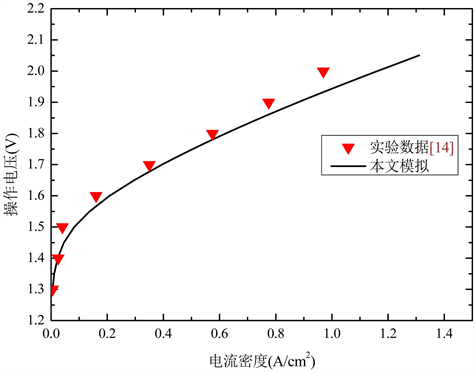Figure 3. Comparison of the simulated PEM electrolyzer cell performance and the experimental data  , T = 363.15 K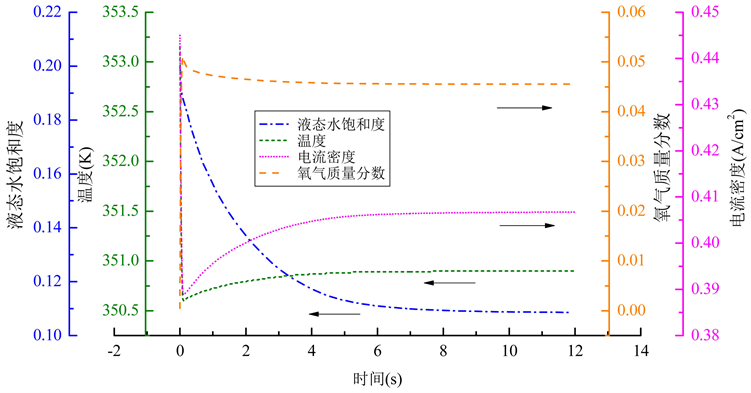Figure 4. Dynamic responses of relevant parameters in the central point of the anode catalyst layer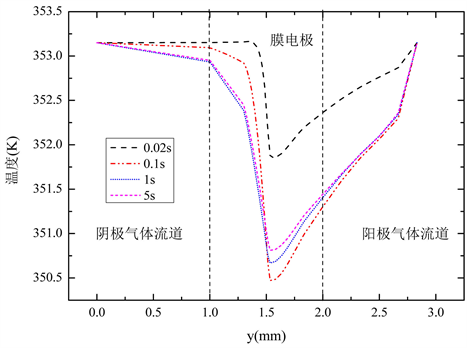Figure 7. Temperature distribution along line B-B at different times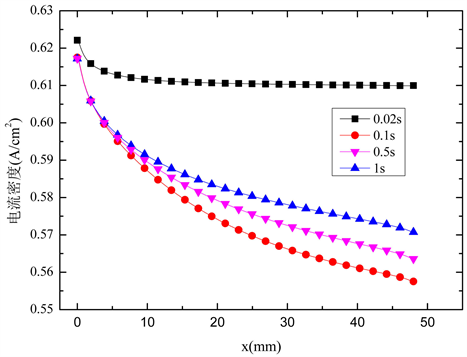Figure 8. Current density distribution along the catalyst layer central line at different times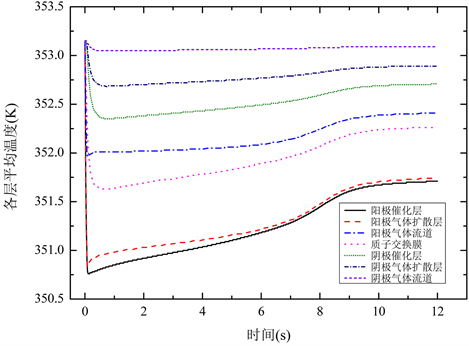Figure 10. Transient responses of the average temperature in different layers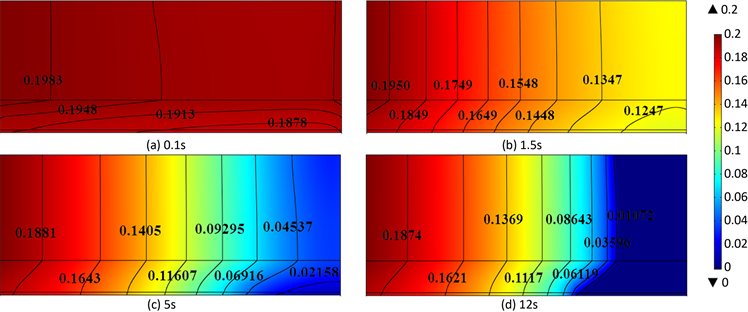Figure 11. Transient responses of the average temperature in different layers

4. 结论

1) 液态水饱和度、温度、电流密度、质量分数的瞬态响应规律是不同的；液态水从瞬态到达稳态的时间较温度、电流密度、质量分数到达稳态的时间长。

2) 液态水在多孔介质内的变化梯度较流道内的变化梯度大；靠近流道入口处截面上，液态水饱和度的变化梯度较出口处截面上的变化梯度小。液态水饱和度在沿流道进口至出口方向和气体流道至催化层方向均逐渐减小，且在催化层内液态水饱和度值最小。

3) 电解池的总体温度是下降的，且电池的最低温度始终出现在阳极催化层中；阳极区域内温度的变化梯度较阴极区域内温度的变化梯度大。电池的最低温度在0.1 s时出现了最小值，此时电池的总体温度降低了3 K左右。

4) 氢气质量分数在靠近流道入口处截面上的变化梯度较出口处截面上的变化梯度小；氢气质量分数沿流道进口至出口方向和气体流道至催化层方向均逐渐增大，且在催化层内氢气质量分数最大。

  程俊, 叶芳, 张伟, 等. 储能用质子交换膜电解池的发展现状[J]. 化学与生物工程, 2015(1): 1-7.  Guo, Q., Ye, F., Guo, H., et al. (2017) Gas/Water and Heat Management of PEM-Based Fuel Cell and Electrolyzer Systems for Space Applications. Microgravity Science & Technology, 29, 1-15. https://doi.org/10.1007/s12217-016-9525-6  Xiao, H., Dai, L.Y., Song, J., et al. (2017) Dynamic Response of a Unitized Regenerative Fuel Cell under Various Ways of Mode Switching. International Journal of Energy Research, 3, 1-10. https://doi.org/10.1002/er.3934  Xiao, H., Guo, H., Ye, F., et al. (2016) Numerical Study of the Dynamic Response of Heat and Mass Transfer to Operation Mode Switching of a Unitized Regenerative Fuel Cell. Energies, 9, 1015. https://doi.org/10.3390/en9121015  Wang, L.L., Guo, H., Ye, F., et al. (2016) Two-Dimensional Simulation of Mass Transfer in Unitized Regenerative Fuel Cells under Operation Mode Switching. Energies, 9, 47. https://doi.org/10.3390/en9010047  王昭毅. 质子交换膜电解池两相传质及性能的研究[D]: [硕士学位论文]. 北京: 北京工业大学, 2014.  赵金钢. 燃料电池和电解池内部两相流的数值模拟[D]: [硕士学位论文]. 北京: 北京工业大学, 2010.  Nie, J. and Chen, Y. (2010) Numerical Modeling of Three-Dimensional Two-Phase Gas-Liquid Flow in the Flow Field Plate of a PEM Electrolysis Cell. International Journal of Hydrogen Energy, 35, 3183-3197. https://doi.org/10.1016/j.ijhydene.2010.01.050  Han, B., Mo, J., Kang, Z., et al. (2017) Modeling of Two-Phase Transport in Proton Exchange Membrane Electrolyzer Cells for Hydrogen Energy. International Journal of Hydrogen Energy, 42, 4478-4489. https://doi.org/10.1016/j.ijhydene.2016.12.103  Aubras, F., Deseure, J., Kadjo, J.J.A., et al. (2017) Two-Dimensional Model of Low-Pressure PEM Electrolyser: Two-Phase Flow Regime, Electrochemical Modelling and Experimental Validation. International Journal of Hydrogen Energy, 42, 26203-26216. https://doi.org/10.1016/j.ijhydene.2017.08.211  Cao, T.F., Lin, H., Chen, L., et al. (2013) Numerical Investigation of the Coupled Water and Thermal Management in PEM Fuel Cell. Applied Energy, 112, 1115-1125. https://doi.org/10.1016/j.apenergy.2013.02.031  Lei, X., Liu, X., Alaje, T., et al. (2014) A Two-Phase Flow and Non-Isothermal Agglomerate Model for a Proton Exchange Membrane (PEM) Fuel Cell. Energy, 73, 618-634. https://doi.org/10.1016/j.energy.2014.06.065  Carmo, M., Fritz, D.L., Mergel, J., et al. (2013) A Comprehensive Review on PEM Water Electrolysis. International Journal of Hydrogen Energy, 38, 4901-4934. https://doi.org/10.1016/j.ijhydene.2013.01.151  Mitlitsky, F., Myers, B., Weisberg, A.H., et al. (1999) Reversible (Unitised) PEM Fuel Cell Devices. Fuel Cells Bulletin, 2, 6-11. https://doi.org/10.1016/S1464-2859(00)80110-8  张亚, 朱春玲. 质子交换膜燃料电池二维全电池两相流综合数值模型[J]. 化工学报, 2008, 59(1): 173-181.  Meng, H. (2007) A Two-Phase Non-Isothermal Mixed-Domain PEM Fuel Cell Model and Its Application to Two-Dimensional Simulations. Journal of Power Sources, 168, 218-228. https://doi.org/10.1016/j.jpowsour.2007.03.012  Zamel, N. and Li, X.G. (2010) A Parametric Study of Multi-Phase and Multi-Species Transport in the Cathode of PEM Fuel Cells. International Journal of Energy Research, 32, 698-721. https://doi.org/10.1002/er.1384